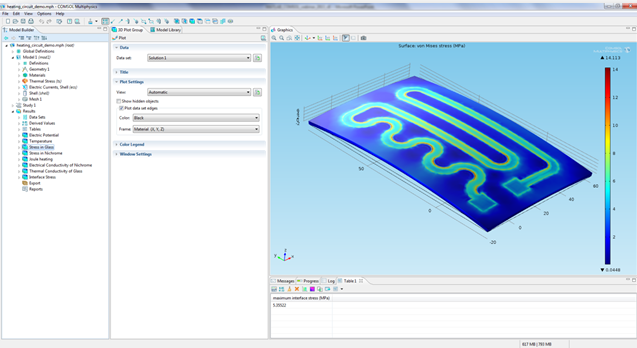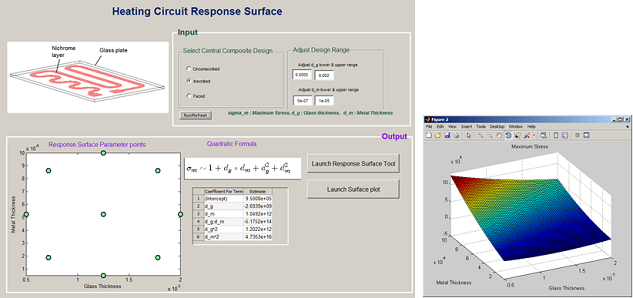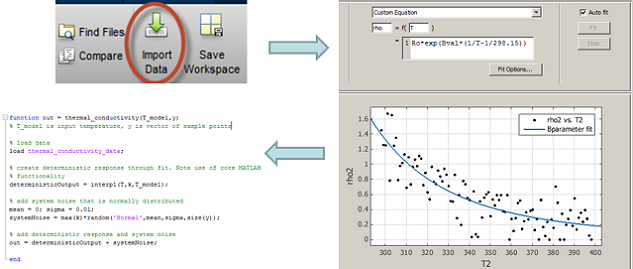# Combining COMSOL Multiphysics® and MATLAB®

June 13, 2013

Did you know that you can combine the strengths of COMSOL Multiphysics® and MATLAB® in real time to solve engineering simulations? To me, that sounds like getting the best of both worlds. Let me explain.

### Why use COMSOL Multiphysics and MATLAB® together?

MATLAB® provides us with an interactive development environment, where you can use a high-level language as well as built-in features to perform data analysis and visualization, numerical computation, algorithm development, and application development. COMSOL Multiphysics, on the other hand, allows you to customize and combine any number of physics, the way you want to. With MATLAB’s open-ended technical computing environment and COMSOL’s multiphysics modeling platform, you could take on almost any scientific challenge.

Most engineering problems revolve around finding optimal design parameters or operating conditions for a device. The device itself functions based on certain principles of physics (or even multiple physics). Let’s use an example. Say, you want to design an electrical heater that has a thin metallic layer deposited on top of glass. The heater works based on the principle of Joule heating, wherein a current passed through the metallic layer produces heat in the metallic layer, which then in turn creates a spatially varying temperature profile in both the metal and the glass layers. As a design engineer, you might want to ensure that the stress generated due to thermal expansion at the interface of glass and metal is not too high to cause mechanical failure of the device. You also know that from a practical stand-point, you can control the thickness of the glass and metallic layers within the dimension limits provided by the fabrication specification. So how can you use COMSOL and MATLAB® to help you solve this design problem?

### How to Combine COMSOL and MATLAB®

You could set up the multiphysics simulation that involves Joule heating and thermal expansion in COMSOL Multiphysics, and perform Design of Experiments (DOE) on the model using functionality that is available as part of the Statistics Toolbox™ in MATLAB®.You can certainly write a MATLAB® script that creates Response Surface Designs (a DOE technique) for your COMSOL model. Or if you’re interested in easy repeated interactions with your model, or would like to share your work with others, you could write a custom MATLAB® app such as the one shown below. The app shown here can solve the COMSOL model recursively for a set of design points, and create a response surface that you or a decision-maker could then use to make an educated engineering decision.Heating Circuit response surface.

To make the simulation more realistic, you could even use experimental data that describes the variation in material properties as a function of temperature. Such numerical data could be pre-processed using MATLAB’s core functions or via specialized functions/apps available in toolboxes such as the Curve Fitting Toolbox™, and you can easily call those functions from within the COMSOL model.Now you have a MATLAB® function describing the material behavior that is called by a COMSOL Multiphysics model, which in turn is called by a MATLAB® Design of Experiments app. You can’t ask for a tighter integration between the two software than this, can you?

### Integrate with LiveLink™ for MATLAB®

In order to combine and use COMSOL Multiphysics together with MATLAB®, you will need LiveLink™ for MATLAB®. This interfacing tool allows you to create a bidirectional integration of COMSOL Multiphysics and MATLAB®. Besides solving the type of problem described above, you can perform a host of advanced modeling operations as a result of the flexibility provided by the interfacing tool. Below, I have listed some of its key benefits:

• Using functions and variables
• Call built-in and user-defined MATLAB® function from COMSOL Desktop
• Call COMSOL model as a function in MATLAB® workspace
• Communication between COMSOL and MATLAB® variables
• Programmatic control for setting up and running models
• Repetitive operations using for-loop and while-loop
• Controls using if-else, switch, break, and continue
• Data extraction and analysis
• Customized data extraction and statistical analysis
• State-space matrix for system level modeling

For more information on how to work with COMSOL Multiphysics and MATLAB® together, check out the archived version of our webinar on Using MATLAB® with COMSOL Multiphysics® to Simulate and Customize Designs. In this webinar, Deepak Ramaswamy from The MathWorks and I will take you through a number of simulation scenarios where the combined strengths of COMSOL Multiphysics and MATLAB® can turn out to be advantageous. You will also see a series of short tutorial videos showing the details of setting up and solving the design optimization problem of the heating circuit.

MATLAB is a registered trademark of The MathWorks Inc.

#### Categories##### Yu Zhang
July 20, 2018

Hi, Supratik Datta,

Thank you for sharing us this useful blog. Could you please do me a favor. I have a problem about using external functions of MATLAB in COMSOL. For most case, the output of the function is a single value. But now I want to get a elasticity matrix from the external function which has six components. Do you mind sharing me some ideas about that? Thank you so much for your help.

Best regards,

Yu##### Lars Gregersen
August 8, 2018

Hi Yu

Unfortunately, there are no good ideas. Comsol only allows functions that return a scalar.

Hence you need 6 independent function files. Since these functions most likely are related and expensive you can sometimes cache calculations (e.g. using persistent variables) and return the correct results when requested. This is not easy to make – and hard to do efficiently.

I would suggest that you write to support@comsol.com and include your model with a description of you desired usage. Then someone can decide if this is something we can improve upon.

EXPLORE COMSOL BLOG